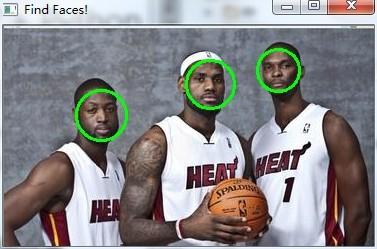# 手把手教你如何用 OpenCV + Python 實現人臉檢測

## 必備知識

Haar-like

Haar-like百科釋義。通俗的來講，就是作為人臉特征即可。

Haar特征值反映了圖像的灰度變化情況。例如：臉部的一些特征能由矩形特征簡單的描述，如：眼睛要比臉頰顏色要深，鼻梁兩側比鼻梁顏色要深，嘴巴比周圍顏色要深等。

opencv api

import cv2
image = cv2.imread(imagepath)

import cv2
gray = cv2.cvtColor(image,cv2.COLOR_BGR2GRAY)

opencv 的強大之處的一個體現就是其可以對圖片進行任意編輯，處理。

import cv2
cv2.rectangle(image,(x,y),(x+w,y+w),(0,255,0),2)

import cv2
cv2.imshow("Image Title",image)

import cv2
face_cascade = cv2.CascadeClassifier(r'./haarcascade_frontalface_default.xml')

import cv2

# 探測圖片中的人臉

gray,
scaleFactor = 1.15,
minNeighbors = 5,
minSize = (5,5),
flags = cv2.cv.CV_HAAR_SCALE_IMAGE
)

import cv2

print "發現{0}個人臉!".format(len(faces))

for(x,y,w,h) in faces:
cv2.rectangle(image,(x,y),(x+w,y+w),(0,255,0),2)

## 實例# coding:utf-8
import sys

sys.setdefaultencoding('utf8')
#    __author__ = '郭 璞'
#    __date__ = '2016/9/5'
#    __Desc__ = 人臉檢測小例子，以圓圈圈出人臉
import cv2
# 待檢測的圖片路徑
imagepath = r'./heat.jpg'

# 獲取訓練好的人臉的參數數據，這里直接從GitHub上使用默認值

# 讀取圖片
gray = cv2.cvtColor(image,cv2.COLOR_BGR2GRAY)

# 探測圖片中的人臉
gray,
scaleFactor = 1.15,
minNeighbors = 5,
minSize = (5,5),
flags = cv2.cv.CV_HAAR_SCALE_IMAGE
)

print "發現{0}個人臉!".format(len(faces))

for(x,y,w,h) in faces:
# cv2.rectangle(image,(x,y),(x+w,y+w),(0,255,0),2)
cv2.circle(image,((x+x+w)/2,(y+y+h)/2),w/2,(0,255,0),2)

cv2.imshow("Find Faces!",image)
cv2.waitKey(0)D:\Software\Python2\python.exe E:/Code/Python/DataStructor/opencv/Demo.py

## 總結

posted @ 2019-12-05 17:25  pypypypy  閱讀(...)  評論(...編輯  收藏